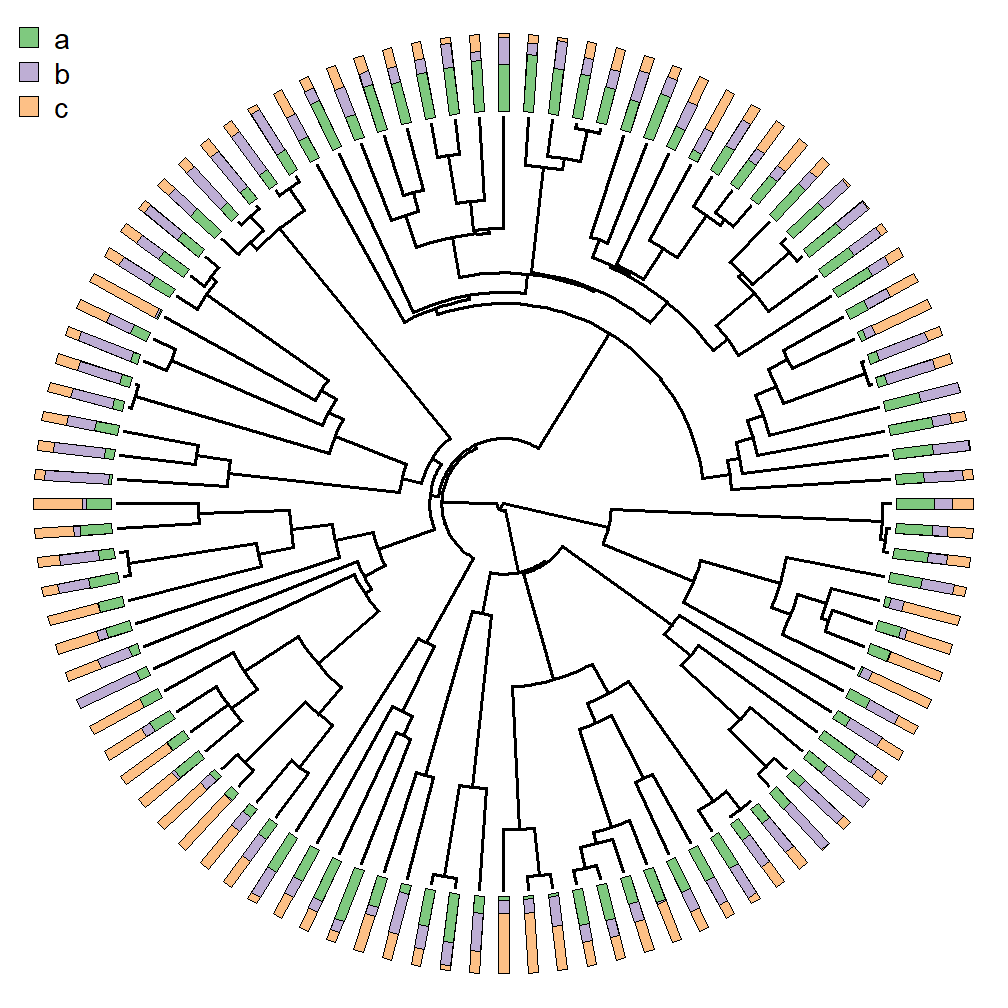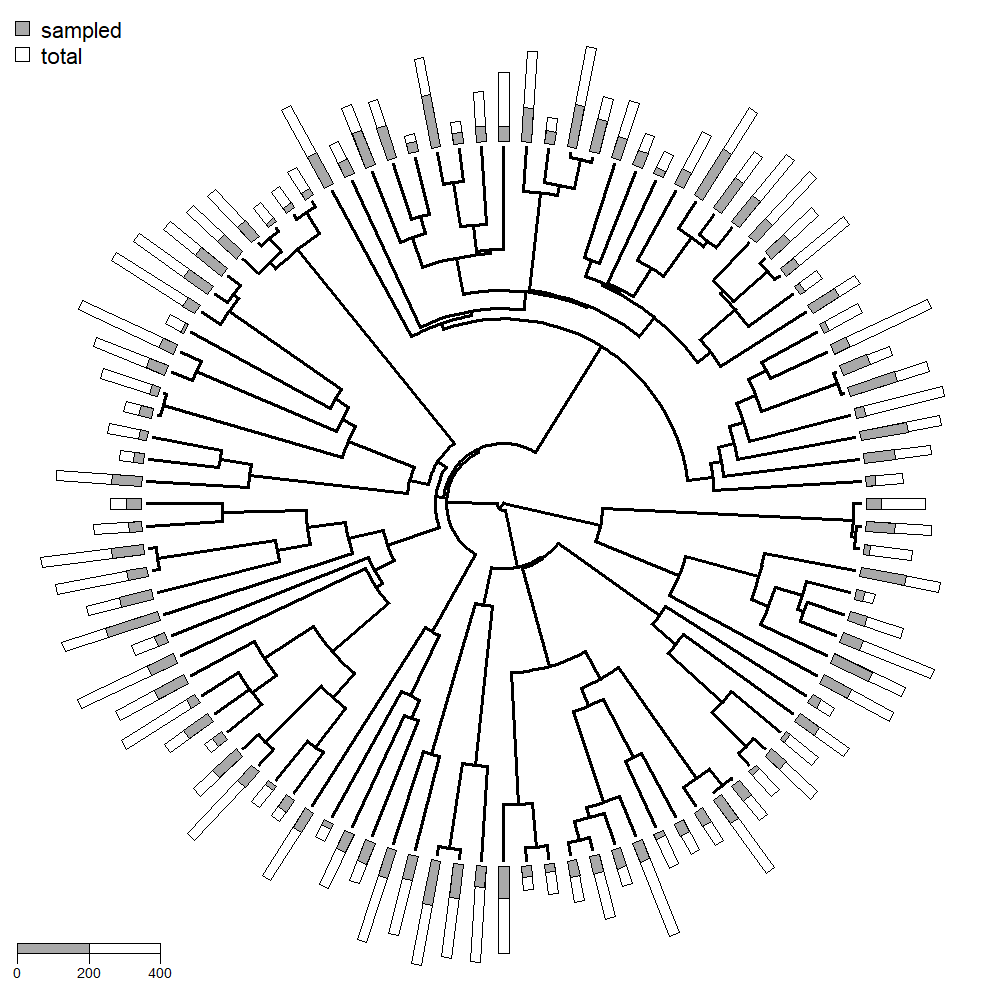## Saturday, April 7, 2018

### Update & hack to get stacked bars in `plotTree.wBars`

A little while ago I received the following user request:

“I really like your `plotTree.wBars` function in phytools, but I'm interested in a slight modification. I'd like to use it to show the proportion of each genus for which I have data about a particular trait. So the length of the bar would be proportional to the size of that genus, and then I'd like to color in the bar to indicate how many species have been evaluated (e.g., [Genus A] has ~2500 species, and I have data for roughly 100, so the bar would be quite long, but 1/25 would be shaded in).”

Firstly, phytools already has another function, `plotTree.barplot`, that can easily plot stacked barplots next to a plotted tree. Here is a nice example, but there are others on my blog.

`plotTree.barplot` only works for right facing trees. One of the nice things about `plotTree.wBars` is that it can work with fan-style (i.e., circular) trees too. Unfortunately, stacked bar plots are not explicitly supported.

I just pushed a tiny update, however, that enables the following hack.

First, let's imagine that our data consist of proportions for each species summing to 1.0:

``````library(phytools)
tree
``````
``````##
## Phylogenetic tree with 100 tips and 99 internal nodes.
##
## Tip labels:
##  t10, t12, t17, t19, t95, t96, ...
##
## Rooted; includes branch lengths.
``````
``````head(X)
``````
``````##             a         b           c
## t10 0.3611411 0.5059744 0.132884464
## t12 0.5133296 0.4712203 0.015450042
## t17 0.5612333 0.2360284 0.202738273
## t19 0.4778141 0.5181375 0.004048438
## t95 0.1104973 0.6544446 0.235058096
## t96 0.1234555 0.6716241 0.204920351
``````

The next step is that we want to calculate the cumulative sums for each row. We can do this as follows:

``````Y<-t(apply(X,1,cumsum))
``````
``````##             a         b c
## t10 0.3611411 0.8671155 1
## t12 0.5133296 0.9845500 1
## t17 0.5612333 0.7972617 1
## t19 0.4778141 0.9959516 1
## t95 0.1104973 0.7649419 1
## t96 0.1234555 0.7950796 1
``````

Now let's plot:

``````library(RColorBrewer)
cols<-brewer.pal(n=ncol(Y),"Accent")
scale<-0.2*max(nodeHeights(tree))
plotTree.wBars(tree,Y[,ncol(Y)],type="fan",scale=scale,col=cols[ncol(Y)])
obj<-get("last_plot.phylo", envir = .PlotPhyloEnv)
for(i in (ncol(Y)-1):1){
col=cols[i])
}
legend(x="topleft",legend=colnames(Y),pch=22,
pt.cex=2,pt.bg=cols,cex=1.2,bty="n",horiz=FALSE)
``````To the original question, our stacked bars do not need to represent proportions. Let's imagine (as in the question) that we have a matrix containing two columns: the first the number of lineages sampled from a clade, and the second the total diversity.

E.g.:

``````head(Z)
``````
``````##     sampled total
## t10      26   104
## t12      87   190
## t17     133   227
## t19      28   254
## t95     137   231
## t96      82   149
``````

This time we do not need to compute the cumulative sum of the two columns as this is effectively what `total"` gives us.

``````cols<-c("darkgrey","white")
scale<-0.3*max(nodeHeights(tree))/max(Z)
plotTree.wBars(tree,Z[,ncol(Z)],type="fan",scale=scale,col=cols[ncol(Z)])
obj<-get("last_plot.phylo", envir = .PlotPhyloEnv)
col=cols)
obj<-legend(x="topleft",legend=colnames(Z),pch=22,
pt.cex=1.5,pt.bg=cols,cex=0.9,bty="n",horiz=FALSE)
leg.length<-400 ## legend length
polygon(0.97*obj\$rect\$left+c(0,0,leg.length*scale,
leg.length*scale),-obj\$text\$y+c(0,
diff(par()\$usr[3:4])/100,diff(par()\$usr[3:4])/100,0))
polygon(0.97*obj\$rect\$left+c(0,0,leg.length*scale/2,
leg.length*scale/2),-obj\$text\$y+c(0,
diff(par()\$usr[3:4])/100,diff(par()\$usr[3:4])/100,0),
col="darkgrey")
for(i in 0:2){
lines(rep(0.97*obj\$rect\$left+i/2*leg.length*scale,2),
-obj\$text\$y+c(0,-diff(par()\$usr[3:4])/100))
text(0.97*obj\$rect\$left+i/2*leg.length*scale,-obj\$text\$y,
i*leg.length/2,cex=0.6,pos=1)
}
``````Cool.

To make this work you will need to update phytools from GitHub:

``````library(devtools)
install_github("liamrevell/phytools")
``````

FYI, the data for this demo were simulated as follows:

``````tree<-pbtree(n=100,scale=1)
X<-fastBM(tree,nsim=3,bounds=c(0,Inf))
X<-t(apply(X,1,function(x) x<-x/sum(x)))
colnames(X)<-c("a","b","c")
total<-setNames(round(runif(n=Ntip(tree),min=50,max=300)),tree\$tip.label)
sampled<-round(runif(n=Ntip(tree),min=0.1,max=0.6)*total)
Z<-cbind(sampled,total)
``````